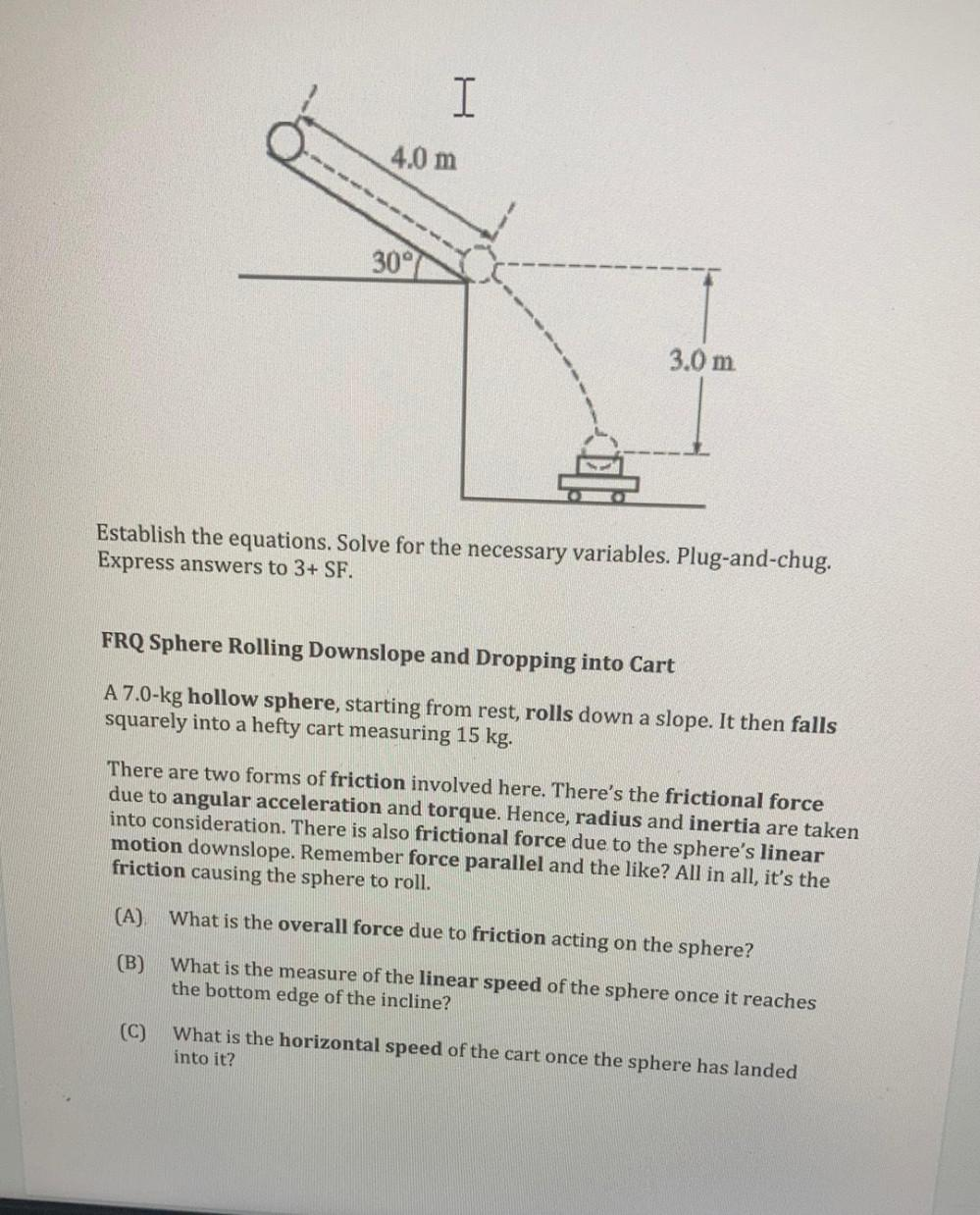Question:

# I 4.0 m 30° 3.0 m Establish the equations. Solve for the necessary variables. Plug-and-chug. Express answers to 3+ SF. FRQ SpherI 4.0 m 30° 3.0 m Establish the equations. Solve for the necessary variables. Plug-and-chug. Express answers to 3+ SF. FRQ Sphere Rolling Downslope and Dropping into Cart A 7.0-kg hollow sphere, starting from rest, rolls down a slope. It then falls squarely into a hefty cart measuring 15 kg. There are two forms of friction involved here. There's the frictional force due to angular acceleration and torque. Hence, radius and inertia are taken into consideration. There is also frictional force due to the sphere's linear motion downslope. Remember force parallel and the like? All in all, it's the friction causing the sphere to roll. (A) What is the overall force due to friction acting on the sphere? (B) What is the measure of the linear speed of the sphere once it reaches the bottom edge of the incline? (C) What is the horizontal speed of the cart once the sphere has landed into it?